# HDL Implementation of WLAN Receiver

This example shows how to design a wireless local area network (WLAN) receiver that can recover signal and data field information from a WLAN signal. This example supports both binary convolutional coding (BCC) and low-density parity-check (LDPC) channel coding techniques. The Simulink® model in this example is optimized for HDL code generation and hardware implementation.

The example supports single-input single-output (SISO) orthogonal frequency division multiplexing (OFDM) modulation, 20 MHz bandwidth option for non-high-throughput (non-HT), high-throughput (HT), and very-high-throughput (VHT) frame formats and 40 MHz bandwidth option for high-throughput (HT) and very-high-throughput (VHT) frame formats for BCC channel coding. The example supports 20 MHz and 40 MHz bandwidth options for HT and VHT frame formats for LDPC channel coding. You can select the channel coding type from the `Input Generation` subsystem. For more information on the WLAN frame formats and frame structure, see WLAN PPDU Structure (WLAN Toolbox). The block diagram shows the high-level overview of a WLAN receiver design. The WLAN HDL Time and Frequency Synchronization explains the functionality of the `Time and Frequency Synchronization` subsystem. This subsystem accepts Rx input waveform and outputs time and frequency synchronized waveform.

To design a WLAN receiver, along with the `Time and Frequency Synchronization` subsystem, the model requires a few more blocks as shown in the block diagram.The OFDM Demodulation block converts the time-domain signal to frequency-domain subcarriers. The Channel Estimator block uses demodulated legacy long training fields (L-LTFs) of a WLAN signal to estimate the channel frequency response. To equalize the pilot and data subcarriers of non-HT portion of the WLAN signal, the channel equalizer uses the estimated channel frequency response. The non-HT portion of the WLAN signal includes legacy SIGNAL(L-SIG) field, high-throughput SIGNAL fields 1 and 2 (HT-SIG 1 and 2), very-high-throughput SIGNAL fields A and B (VHT-SIG-A and VHT-SIG-B) and legacy Data field. Similarly, the channel is estimated using the demodulated HT or VHT LTFs of a WLAN signal to equalize the pilot and data subcarriers of HT or VHT portion of the WLAN signal. The HT or VHT portion of the WLAN signal includes VHT-SIG-B, HT-Data, and VHT-Data.

After equalization, non-HT common phase error (CPE) estimation is performed using non-HT pilots. The estimated CPE is used to correct data subcarriers of the non-HT portion of the WLAN signal. Similarly, HT or VHT common phase error (CPE) estimation is performed using HT or VHT pilots. The estimated CPE is used to correct data subcarriers of the HT or VHT portion of the WLAN signal. Common phase noise error corrected data is used for frame format detection, signal, and Data field recovery.

Frame format detector and SIGNAL field recovery detects the frame format between non-HT, HT, and VHT frames and decodes the transmitted bits from WLAN signal fields L-SIG, HT-SIG 1 and 2 and VHT-SIG-A 1 and 2. If the detected frame format is non-HT, the frame format controller passes non-HT CPE corrected data to Data recovery. Alternatively, if the detected frame format is HT or VHT, the frame format controller passes HT or VHT CPE corrected data to Data recovery. Data recovery decodes the transmitted bits from WLAN data fields L-Data, HT-Data and VHT-Data using signal parameters such as modulation and coding scheme (MCS), physical layer convergence protocol service data unit (PSDU) length, and the channel coding type. VHT-SIG-B is also decoded as part of Data recovery for the VHT frame. The example validates the Simulink® WLAN receiver model output by using MATLAB® functions in WLAN Toolbox™.

### Model Architecture

Open the `wlanhdlReceiver` model to run the example. This figure shows the high-level overview of a WLAN receiver model.

```modelname = 'wlanhdlReceiver'; open_system(modelname); ```Time and Frequency Synchronization

The `Time and frequency synchronization` subsystem performs receiver filtering and coarse time and frequency estimation and corrections on the filtered signal. Then, the subsystem fine tunes the time and frequency estimation and corrections to remove any residual offsets. The `wlanhdlReceiverInit.m` file initializes filter coefficients.

Open the `WLANTimeAndFrequencySynchronization` subsystem to see the synchronization process.

```open_system([modelname '/WLANHDLReceiver/WLANTimeAndFrequencySynchronization'],'force'); ```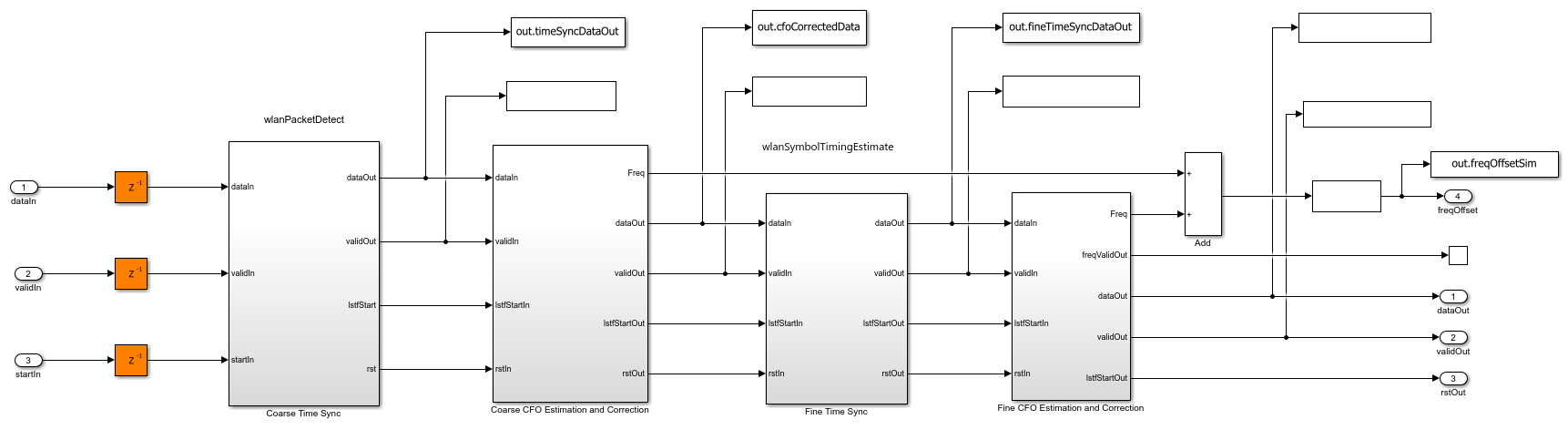OFDM Demodulation

The OFDM Demodulator block converts time-domain signals to frequency-domain subcarriers. The block provides the flexibility to change the orthogonal frequency division multiplexing (OFDM) parameters FFT length, Cyclic prefix length, Number of left guard subcarriers, and Number of right guard subcarriers during the runtime. In this example, based on bandwidth option, the cyclic prefix (CP) length varies for different fields in the WLAN signal. For example, the first symbol of L-LTF uses a CP length of 32 or 64, the second symbol of the L-LTF uses a CP length of 0, and the remaining fields of the WLAN signal uses a CP length of 16 or 32 for 20 MHz and 40 MHz, respectively. In this example, the FFT length parameter is set to `64` for 20 MHz and `128` for 40 MHz and the Number of left guard subcarriers and Number of right guard subcarriers parameters are set to `4` and `3` for 20 MHz and `6` and `5` for 40 MHz, respectively.

```open_system([modelname '/WLANHDLReceiver/OFDMDemodulation']); ```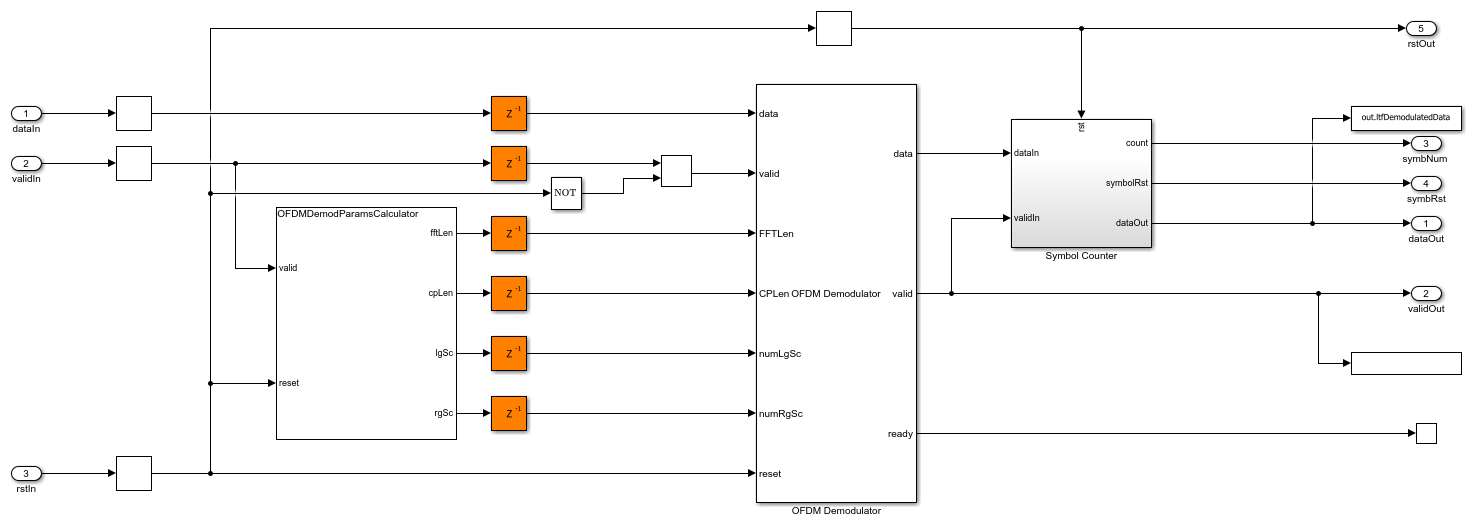The `OFDMDemodulationParameterCalculator` MATLAB function controls the OFDM Demodulator block parameters for different fields of the WLAN packet. The `OFDMDemodulationParameterCalculator` MATLAB function calculates the number of used subcarriers to determine the number of OFDM symbols in the WLAN packet.

Non-HT Channel Estimation and Equalization

The `NonHTChannelEstAndEqualize` subsystem is used for L-LTF channel estimation. The input is given to the OFDM Channel Estimator block. The OFDM Channel Estimator block implements least squares (LS) estimation for the channel estimation and performs averaging on the estimates from two L-LTF symbols of the WLAN signal. The OFDM Equalizer block uses the resultant averaged channel estimate to perform zero forcing (ZF) equalization on data.

```open_system([modelname '/WLANHDLReceiver/NonHTChannelEstAndEqualize']); ```HT or VHT Channel Estimation and Equalization

The `HTorVHTChannelEstAndEqualize` subsystem is similar to the `NonHTChannelEstAndEqualize` subsystem. For a SISO configuration, only one HT or VHT LTF exists, so averaging is disabled in the OFDM Channel Estimator block.

```open_system([modelname '/WLANHDLReceiver/HT_VHTChannelEstAndEqualize']); ```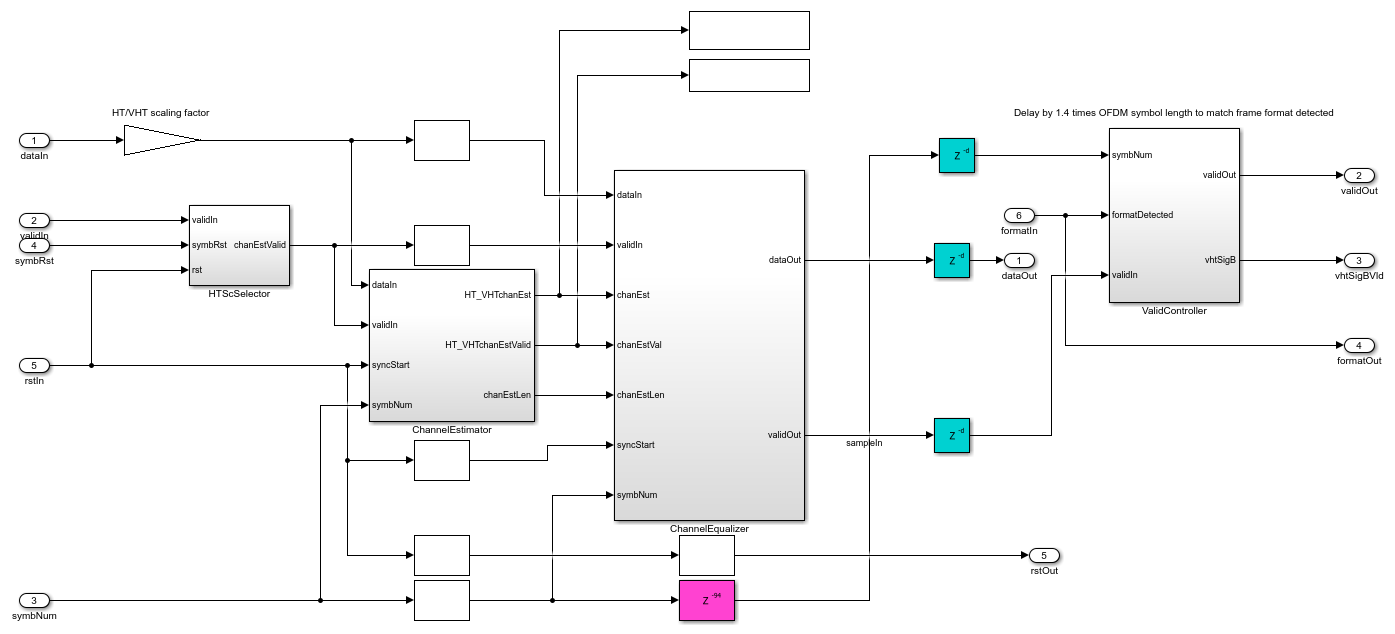Non-HT Common Phase Noise Estimation and Correction

The `NonHTCPEEstAndCorrection` subsystem estimates the common phase noise or residual frequency offset for the non-HT portion of the WLAN signal. CPE estimation requires references such as non-HT pilot positions, a non-HT pilot sequence, and pseudo-noise (PN) sequence as described in Equation 17-25 in [ 1 ]. The `wlanhdlRxinint.m` script initializes these known references and stores them in 1-D lookup tables in the subsystem. The `PolarityGenerator` subsystem gives the polarity of the pilots based on the symbol number. The reference pilots are multiplied with polarity for CPE estimation. The estimated CPE is averaged on all of the pilot subcarriers in an OFDM symbol and is used for the correction of data subcarriers of non-HT portion of the WLAN packet.

```open_system([modelname '/WLANHDLReceiver/NonHTCPEEstAndCorrection']); ```HT or VHT Common Phase Noise Estimation and Correction

The `HTorVHTCPEEstAndCorrect` subsystem is similar to the `NonHTCPEEstAndCorrect` subsystem. This subsystem performs CPE estimation and correction using HT or VHT pilot positions and a HT or VHT pilot sequence.

```open_system([modelname '/WLANHDLReceiver/HT_VHTCPEEstAndCorrection']); ```Frame Format Identification and Controller

To recover the signal and data field information from a WLAN signal, you must use the WLAN receiver frame format. This example supports non-HT, HT, and VHT frame formats. The `FrameFormatDetector` subsystem detects the frame format as non-HT, HT, or VHT by buffering 3 OFDM symbols after L-LTFs, at the output of the `NonHTCPEEstAndCorrect` subsystem. This flow chart describes the frame format detection. For more information, see [ 2 ].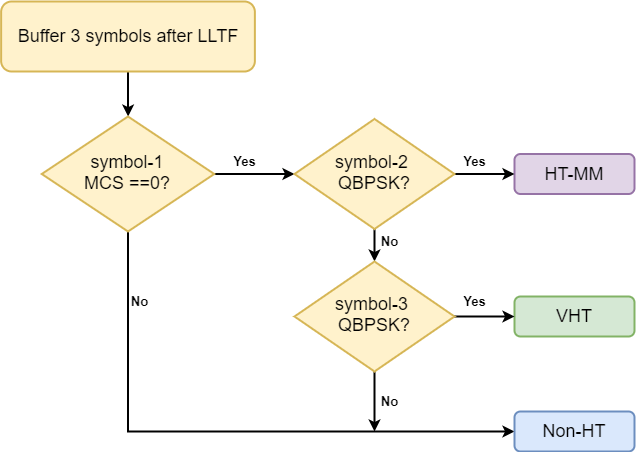The `Signal Recovery` subsystem decodes the MCS from the first symbol L-SIG. If the MCS is not 0, the `FrameFormatDetector` subsystem detects the frame format as non-HT. If the MCS is 0, it checks the modulation scheme of OFDM symbol 2. If the modulation scheme of symbol 2 is QBPSK, the subsystem detects the format as HT. If the modulation scheme of symbol 2 is BPSK, it checks the modulation scheme of OFDM symbol 3. If the modulation scheme of symbol 3 is QBPSK, the subsystem detects the format as VHT. If the modulation scheme of symbol 3 is BPSK, the subsystem detects the format as non-HT.

If the `FrameFormatDetector` subsystem detects frame format as non-HT, then the remaining OFDM symbols, including OFDM symbols 2 and 3, are treated as L-Data. The `FrameFormatController` subsystem passes the output of the `NonHTCPEEstAndCorrect` subsystem to the `DataRecovery` subsystem to decode L-Data.

If the `FrameFormatDetector` subsystem detects the frame format as HT or VHT, the `FrameFormatController` subsystem passes the output of the `HT_VHTCPEEstAndCorrect` subsystem to the `DataRecovery` subsystem to recover HT-Data or VHT-Data.

Signal Recovery

The `SignalRecovery` subsystem recovers the header information to decode data bits from L-SIG, HT-SIG, and VHT-SIG fields. The output of the `NonHTCPEEstAndCorrect` subsystem corresponding to signal fields is streamed into the `SignalRecovery` subsystem. The Symbol Demodulator block performs BPSK and QBPSK soft symbol demodulation on signal fields in the WLAN packet. The channel decoding includes `Deinterleaver` subsystem and Viterbi Decoder block.

The `Deinterleaver` subsystem performs deinterleaving on the symbol demodulated data with a maximum block size of 48 and the number of columns as 16. The Viterbi Decoder block performs 1/2 rate viterbi decoding on deinterleaved data. For more information on the `Deinterleaver` subsystem, see HDL Interleaver and Deinterleaver.

L-SIG uses the parity to check the error in WLAN L-SIG field, whereas 8-bit cyclic redundancy check (CRC) is used to check the error in the WLAN HT-SIG 1 and 2 and VHT-SIG-B field. The General CRC Syndrome Detector HDL Optimized block is used for CRC error detection and `ParityCalculator` subsystem performs parity calculation. If the CRC checksum or parity fails, the signal field recovery returns the status of parity check or CRC (Pass or Fail).

```open_system([modelname '/WLANHDLReceiver/FrameFormatDetectionAndSignalRecovery/SignalBitRecovery']); ```Data Recovery

The `DataRecovery` subsystem uses the WLAN signal fields to decode data bits. The registers are used to store WLAN signal field information. These registers access the WLAN signal field information. The Symbol Demodulator block performs soft-bit BPSK, QPSK, 16-QAM, or 64-QAM symbol demodulation associated with the modulation type retrieved from the WLAN signal field information.

If the decoded WLAN channel coding type is BCC, the `Deinterleaver` and the `BCC Decoder` subsystems perform deinterleaving and Viterbi decoding on the symbol demodulated data. The `Deinterleaver` subsystem consists of different deinterleavers for non-HT and HT or VHT data. The deinterleaver for non-HT data is configured with a block size of 48 and number of columns as 16. The deinterleaver for HT or VHT data is configured with a block size of 52 and number of columns as 13 for 20 MHz and a block size of 216 and number of columns as 18 for 40 MHz, respectively. The `BCC Decoder` subsystem is equipped with Depuncturer and Viterbi Decoder blocks. Each code rate is assigned a predefined punctured vector pattern. Based on the code rate retrieved from the WLAN signal field information, the `BCC Decoder` subsystem performs depuncturing followed by Viterbi decoding.

If the decoded WLAN channel coding is LDPC, the `LDPCToneDemapping` subsystem in the `DataSelector` subsystem performs the LDPC tone demapping for VHT data before symbol demodulation. The `LDPC Decoder` subsystem in this example decodes one LDPC codeword data. The `LDPCParametersCalculator` subsystem computes the required LDPC parameters such as the LDPC length, the number of payload bits, the number of shortened bits, and the number of punctured bits for the codeword. The `LDPC Codeword Formation` subsystem depunctures the symbol demodulated data to form one LDPC codeword using the LDPC parameters. The WLAN LDPC Decoder block decodes the depunctured data based on the codeword block length index and the code rate index.

The decoded bits are streamed through the `Descrambler` subsystem.

```open_system([modelname '/WLANHDLReceiver/DataRecovery']); ```### File structure

This example uses one Simulink models and three MATLAB files.

• `wlanhdlReceiver.slx` — Open the top-level OFDM receiver Simulink model.

• `wlanhdlReceiverInit.m` — This script is initialized in the `InitFcn` callback of the `whdlhdlReceiver.slx`. This script uses `wlanWaveformGenerator.m` to generate the input waveform to the example.

• `wlanhdlRxParameters.m` — Generate the input parameters according to the Standard IEEE 802.11-2016 to run the `wlanhdlReceiver.slx` model. The parameters correspond to non-HT, HT, and VHT frame formats for 20 MHz and 40 MHz bandwidth options.

• `wlanhdlMATLABRxReference.m` — Implement a MATLAB floating-point equivalent WLAN receiver using functions from the WLAN Toolbox.

### Model Inputs and Outputs

The inputs and outputs to the example model are described below

• dataIn — Input data, specified as a complex signed 16-bit signal sampled at 20 Msps for 20 MHz and 40 Msps for 40 MHz bandwidth options.

• validIn — Control signal to validate the dataIn, specified as a Boolean scalar.

• startIn — Control signal to reset the receiver, specified as a Boolean scalar.

• dataOut — Decoded output data bits, returned as a bits.

• validOut — Control signal to validate the dataOut port, returned as a Boolean scalar.

• diagBus — Status signal with diagnostic outputs, returned as a bus signal.

### Verification and Results

This example model accepts a WLAN transmit waveform as an input along with valid and start signals. The model returns decoded information bits as an output along with a valid signal. The `wlanhdlReceiverInit.m` script provides the input to the model. For the demonstration of the example, the `wlanWaveformGenerator.m` function in the script generates the VHT mode, 20 MHz BCC channel coded frame, which is passed through the TGac channel with a delay profile of Model A. The additive white Gaussian noise (AWGN) at a 30 dB signal-to-noise ratio (SNR) is added to the transmit waveform with other channel impairments such as 20 kHz CFO and a timing offset of 256. To verify the example for LDPC channel coding, set the Channel coding parameter to `LDPC` on the `Input Generation` subsystem, and run the example.

```fprintf('\n Simulating WLAN HDL receiver \n'); out = sim(modelname); close_system(modelname,0); fprintf('\n HDL simulation complete. Data decoded. \n'); ```
``` Simulating WLAN HDL receiver HDL simulation complete. Data decoded. ```

Verify the outputs of this example using WLAN Toolbox™ functions. Specify the same input waveform to the Simulink model and to the MATLAB equivalent receiver. Compare the outputs to validate the example.

```fprintf('\n Comparing WLAN MATLAB reference receiver \n') wlanhdlMATLABRxReference; fprintf('\n MATLAB simulation complete. \n'); simOut = squeeze(out.rxBits(out.rxBitsValid)); errSig = (bitxor(logical(psdu),simOut)); err = sum(errSig); hConstData = out.headerConstellation(out.headerConstellationValid); figure; plot(hConstData,'o'); xlabel('In-Phase'); ylabel('Quadrature') title('Equalized Signal Field Constellation'); m = double(max(abs([real(hConstData(:)); imag(hConstData(:))])) * 1.1); axis([-m m -m m]); dConstData = out.dataConstellation(out.dataConstellationValid); figure; plot(dConstData,'o'); xlabel('In-Phase'); ylabel('Quadrature') title('Equalized Data Field Constellation'); m = double(max(abs([real(dConstData(:)); imag(dConstData(:))])) * 1.1); axis([-m m -m m]); figure; plot(errSig); xlabel('Sample Number'); ylabel('Error Magnitude'); legend('Error') title('Error Magnitude Between Simulink and MATLAB WLAN Receiver Output'); if err == 0 fprintf('\n Simulink and MATLAB outputs match \n'); else fprintf('\n Simulink and MATLAB outputs do not match \n'); end ```
``` Comparing WLAN MATLAB reference receiver MATLAB simulation complete. Simulink and MATLAB outputs match ```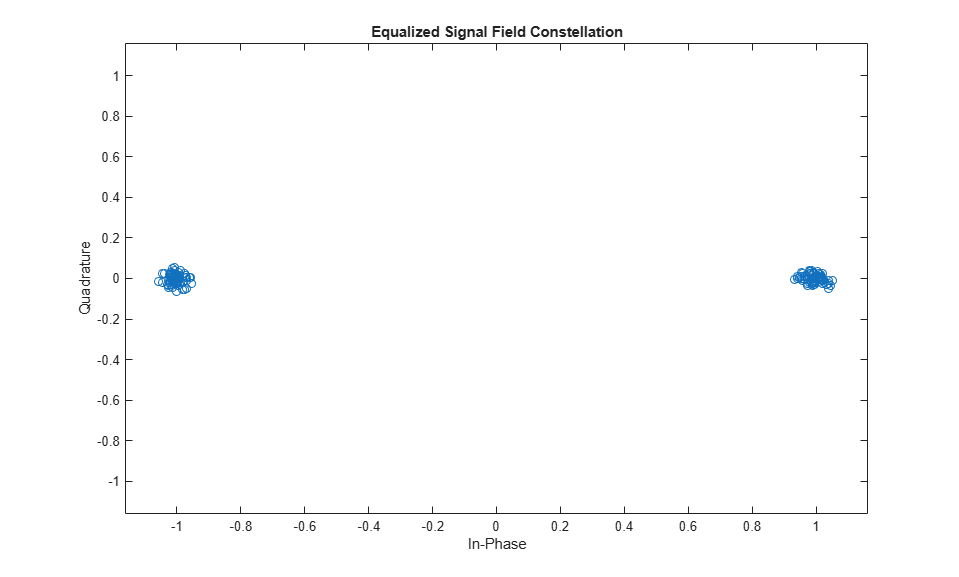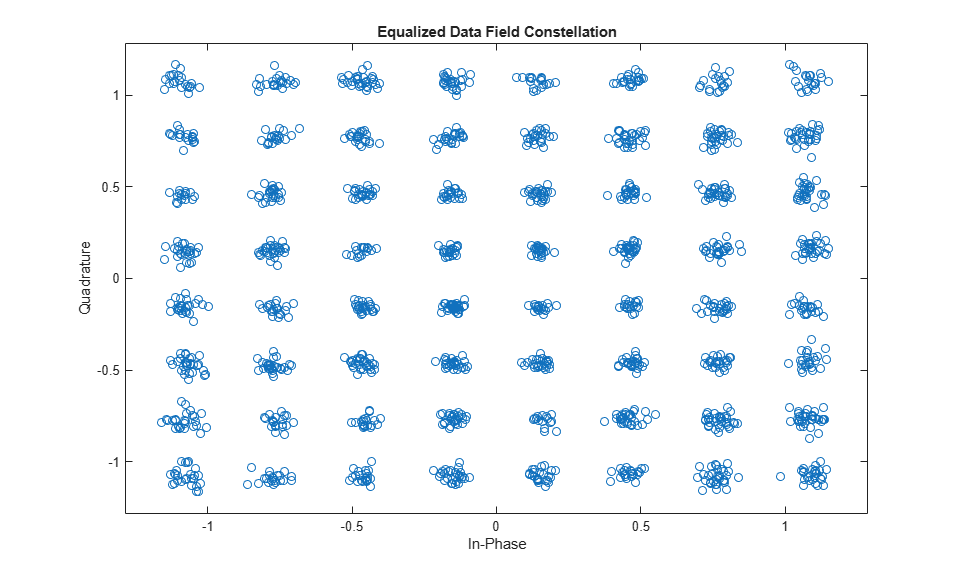### HDL Code Generation and Implementation Results

To generate the HDL code for this example, you must have the HDL Coder™ product. To generate HDL code and an HDL testbench for `WLANHDLReceiver` subsystem, use the `makehdl` and `makehdltb` commands. The resulting HDL code was synthesized for a Xilinx® Zynq® Ultrascale+ RFSoC ZCU111 evaluation board. The table shows the post place and route resource utilization results. The design meets timing with a clock frequency of 306 MHz for 20 MHz and 40 MHz bandwidth options.

```F = table(... categorical({'CLB LUT'; 'CLB Registers'; 'RAMB36'; 'RAMB18';... 'DSP48'}),... categorical({'1,00,805'; '95,245'; '180'; '10'; '323'}),... categorical({'1,03,497'; '97,282'; '183'; '14'; '351'}),... 'VariableNames',... {'Resources','Usage for 20 MHz', 'Usage for 40 MHz'}); disp(F); ```
``` Resources Usage for 20 MHz Usage for 40 MHz _____________ ________________ ________________ CLB LUT 1,00,805 1,03,497 CLB Registers 95,245 97,282 RAMB36 180 183 RAMB18 10 14 DSP48 323 351 ```

### References

1. IEEE 802.11-2016 - IEEE Standard for Information technology--Telecommunications and information exchange between systems Local and metropolitan area networks--Specific requirements - Part 11: Wireless LAN Medium Access Control (MAC) and Physical Layer (PHY) Specifications.

2. Nanda Kishore Chavali, 'System and method for detecting a frame format' (March 2013), US20130077718A1.